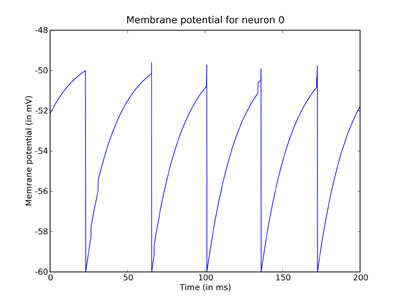# Tutorial 1g: Recording membrane potentials¶

In the previous part of this tutorial, we plotted a raster plot of the firing times of the network. In this tutorial, we introduce a way to record the value of the membrane potential for a neuron during the simulation, and plot it. We continue as before:

```from brian import *

tau = 20 * msecond        # membrane time constant
Vt = -50 * mvolt          # spike threshold
Vr = -60 * mvolt          # reset value
El = -49 * mvolt          # resting potential (same as the reset)
psp = 0.5 * mvolt         # postsynaptic potential size

G = NeuronGroup(N=40, model='dV/dt = -(V-El)/tau : volt',
threshold=Vt, reset=Vr)

C = Connection(G, G)
C.connect_random(sparseness=0.1, weight=psp)
```

This time we won’t record the spikes.

## Recording states¶

Now we introduce a second type of monitor, the `StateMonitor`. The first argument is the group to monitor, and the second is the state variable to monitor. The keyword `record` can be an integer, list or the value `True`. If it is an integer `i`, the monitor will record the state of the variable for neuron `i`. If it’s a list of integers, it will record the states for each neuron in the list. If it’s set to `True` it will record for all the neurons in the group.

```M = StateMonitor(G, 'V', record=0)
```

And then we continue as before:

```G.V = Vr + rand(40) * (Vt - Vr)
```

But this time we run it for a shorter time so we can look at the output in more detail:

```run(200 * msecond)
```

Having run the simulation, we plot the results using the `plot` command from PyLab which has the same syntax as the Matlab `plot`` command, i.e. `plot(xvals,yvals,...)`. The `StateMonitor` monitors the times at which it monitored a value in the array `M.times`, and the values in the array `M`. The notation `M[i]` means the array of values of the monitored state variable for neuron `i`.

In the following lines, we scale the times so that they’re measured in ms and the values so that they’re measured in mV. We also label the plot using PyLab’s `xlabel`, `ylabel` and `title` functions, which again mimic the Matlab equivalents.

```plot(M.times / ms, M / mV)
xlabel('Time (in ms)')
ylabel('Membrane potential (in mV)')
title('Membrane potential for neuron 0')
show()
```You can clearly see the leaky integration exponential decay toward the resting potential, as well as the jumps when a spike was received.